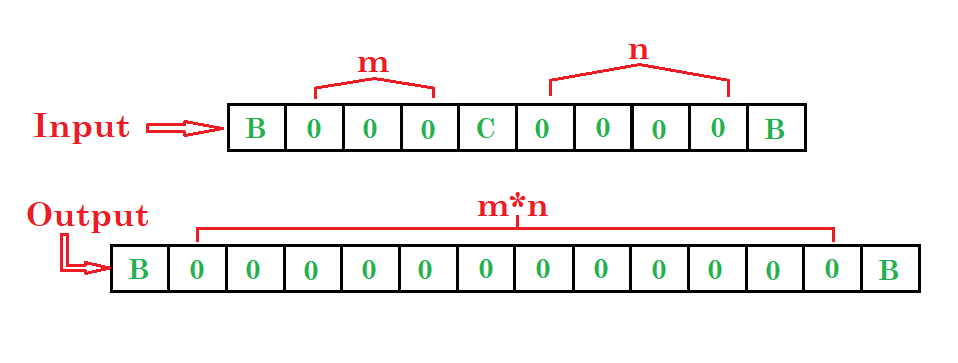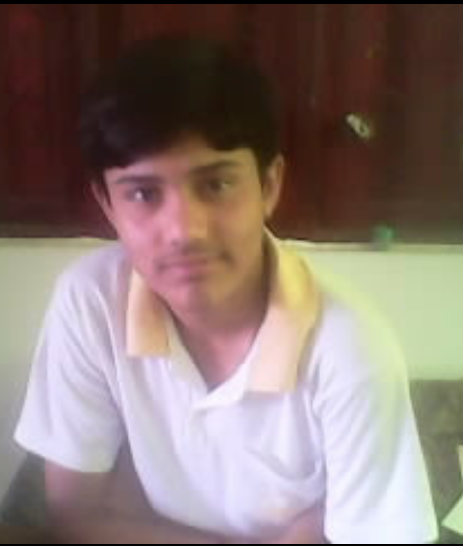# Turing machine for multiplication

Prerequisite – Turing Machine
Problem: Draw a turing machine which multiply two numbers.

Example:Steps:

• Step-1. First ignore 0’s, C and go to right & then if B found convert it into C and go to left.
• Step-2. Then ignore 0’s and go left & then convert C into C and go right.
• Step-3. Then convert all X into X and go right if 0 found convert it into X and go to left otherwise if C found convert it into B and go to right and stop the machine.
• Step-4. If then X found convert it into X and go left then C into C and left then Y into Y and left.
• Step-5. Then if B found convert it into B and right then if Y into 0 and right or if C into C and right and go to step 3 and repeat the process otherwise if 0 found after 4th step then convert it into Y and right then Y into Y and right then C into C and right then 0 into 0 or X into X and right then C into C and right then 0 into 0 and right then B into 0 and left then 0 into 0 and left then C into C and left then 0 into 0 or X into X and left then C into C and left.
• Step-6. Then repeat the 5th step.Here, q0 shows the initial state and q1, q2, ….., q10, q11are the transition states and q12shows the final state.
And X, Y, 0, C are the variables used for multiplication and R, L shows right and left.My Personal Notes arrow_drop_upCheck out this Author's contributed articles.

If you like GeeksforGeeks and would like to contribute, you can also write an article using contribute.geeksforgeeks.org or mail your article to contribute@geeksforgeeks.org. See your article appearing on the GeeksforGeeks main page and help other Geeks.

Please Improve this article if you find anything incorrect by clicking on the "Improve Article" button below.